# Motion Control – How Are the Motor Size, Power Dissipation and Servo Bandwidth Related?

This article discusses how servo loop bandwidth, motor sizing, and power dissipation are related. It provides some background information regarding servo loop gains and bandwidths to set the stage for a discussion of two case studies.

Case 1 is a move and settle application, which shows that servo bandwidth is not related to motor sizing, and Case 2 is a disturbance tracking application, which shows that servo bandwidth directly affects motor sizing. In both cases, power dissipation is the primary driver for sizing a motor.

## Loop Gain, Stability, Bandwidth and Motor Sizing

Servo bandwidth, a direct function of loop gain, includes gains from the plant, the motor, the sensor, the motor drive, and the control gain. Higher loop gain always results in higher bandwidth.

It is possible to increase or decrease loop gain by adjusting the gain of any one component in the loop, such as plant, motor, motor drive, sensor, or control. This means that there is not a single solution of each gain that gives rise to a targeted bandwidth, instead, each gain can be higher or lower, provided that the total gain is conserved.

For instance, the sensor gain for system A could be 1 count for 1 µm and for system B the sensor gain could be 10 counts for 1 µm. The bandwidth of both systems can be the same as long as one of the other gains compensates for the 10x difference. Here, control gain would be the most likely gain to adjust, with system B being 10x less.

Aside from power dissipation, loop gain (and the resulting bandwidth) cannot grow without bound, instead it is limited by stability. Phase margin at the bandwidth frequency and gain margin drives stability when the phase is at -180 degrees.

Higher bandwidths mean that the control system will command the motor to respond at higher frequencies. Higher frequencies lead to higher accelerations and higher required motor forces, all resulting in higher dissipated power.

The allowable bandwidth can be limited by dissipated power (or allowable temperature rise). This means that although stability may allow a system to have a 100 Hz bandwidth, the motor Km and dissipated power requirement selected might limit the bandwidth to be only 50 Hz.

In other words, if 100 Hz is required, the motor Km will be sized accordingly to satisfy the power dissipation requirement.

## Case 1 – Move and Settle

Case 1 shows how motor sizing is not coupled to servo bandwidth, but is coupled to power dissipated in the motor.

The generic block diagram shown in Figure 1 is valid for most of the closed loop control systems. As mentioned before, the loop gain is comprised of the plant gain (Kplant), the motor gain (Kt), the drive gain (Kdrive), the sensor gain (Ksens), and the control gain (Kcntrl).

Kplant, Kdrive, and Kcntrl are highly frequency dependent, but Kt, and Ksens are typically constant (up to a few hundred Hz). If a targeted bandwidth frequency is known, we can solve for Kcntrl at desired bandwidth frequency and confirm stability.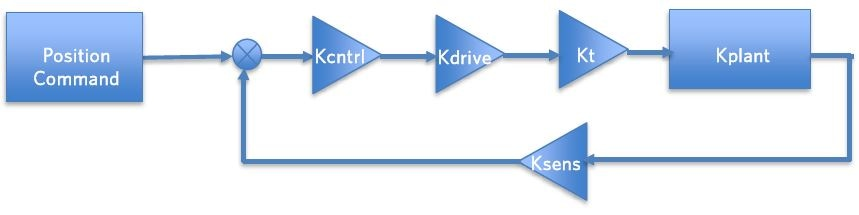Figure 1. System block diagram

In the system depicted in Figure 1, the plant gain is usually a fixed entity. For the sake of this example, let's say the sensor gain is also fixed, and system A and B had a motor gain of 10 N-m/amp and 1 N-m/amp, respectively.

As mentioned earlier, both systems can have the same bandwidth as long as the overall loop gain is the same. In this example, if Kcntrl system B was increased by 10x, system A and B would have the same loop gain, and therefore, the same servo bandwidth.

This example is intended to demonstrate that there is not a unique motor gain that provides a desired servo bandwidth. However, there is a unique torque needed to move the load, given the inertia and acceleration of the load.

Changing the motor gain (N-m/amp) between system A and B means that the current flowing through the motor for system B is 10x larger than that of system A in order to conserve the torque created by the motors. This also reveals that the dissipated power of system B will be higher than system A, and that system B will have a higher coil temperature.

When a load is moved from one location to another, the servo bandwidth does not have a significant impact on the motion trajectory. Figure 2 illustrates how different servo bandwidths will produce very similar motion trajectories. From Figure 2, it is clear that the 100 Hz servo follows the commanded position much better than the 50 Hz; however, the dominant acceleration is created from the trajectory itself.

The acceleration, along with the mass or inertia of the load, and the duty cycle of the movement, all influence the dissipated power (Pd).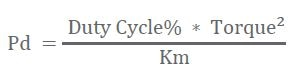Where Duty Cycle is the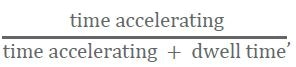Km is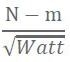and

Torque = inertia (N-m^2) * acceleration (rad/.s^2).

If an allowable Pd is known, we can solve for a Km value and motor sizing is now complete. Motor current and voltage can be traded by the motor design team to arrive at the optimal Kt, depending on the available bus voltage.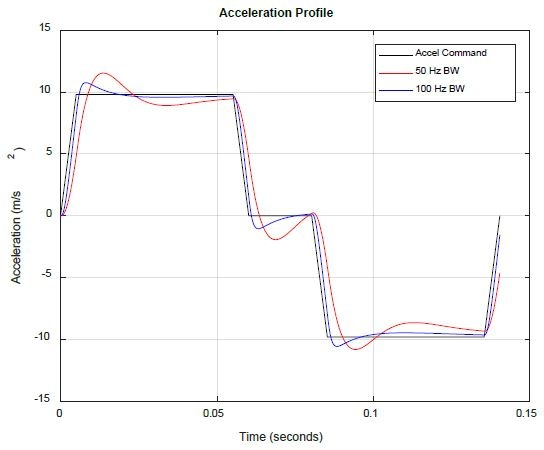Figure 2. Motion trajectory (Black - Commanded, Red - 50 Hz, Green - 100 Hz))

## Case 2 – Disturbance Tracking

In cases where the control system is responding to broadband energy (trying to hold focus, velocity, or position), motor constant Km may limit the servo bandwidth. As the units of the motor constant state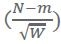, Km is a measure of the ability of a motor to make torque per the square root of dissipated power.

Figure 3 displays a system where the control system is tasked to lower focus error between M2 and the optical structure, while M1 is subjected to a disturbance force, creating motion Z1(t). In this example, the input spectrum Z1(t) has broadband energy up to the kHz frequency range.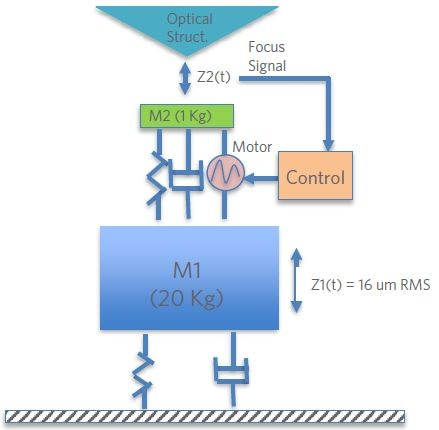Figure 3. Simple disturbance tracking system

The plant and loop transfer functions for the system are shown in Figure 4. The loop is showing ~50 Hz 0 dB crossing and this frequency is called the “servo bandwidth”. A plot of motor force for the 50 Hz case and also the 100 Hz 0 dB case is shown in Figure 5, illustrating how the bandwidth dramatically influences the amplitude of the motor force signal. Dissipated power in the system is: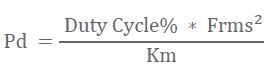Where, Km is the motor constant in (N/√W) and Duty Cycle is 100%. Km is determined using the following expression: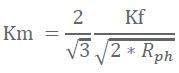In this example, Km is found to be 29 N/√W, where Kf = 50 N/amp, and Rphase = 2 ohms. The dissipated power is 12 watts for the 50 Hz case and 75 watts for the 100 Hz case, clearly revealing that the higher bandwidth case needs more power (6x increase) to track the higher frequencies.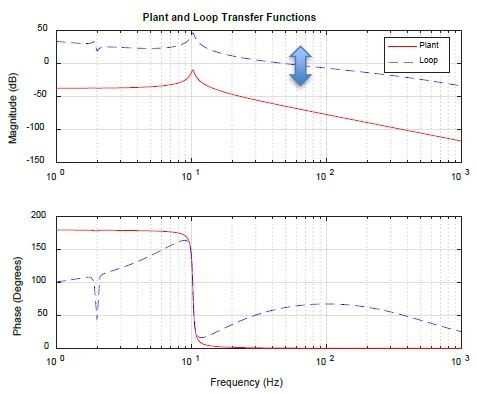Figure 4. Plant and loop transfer functions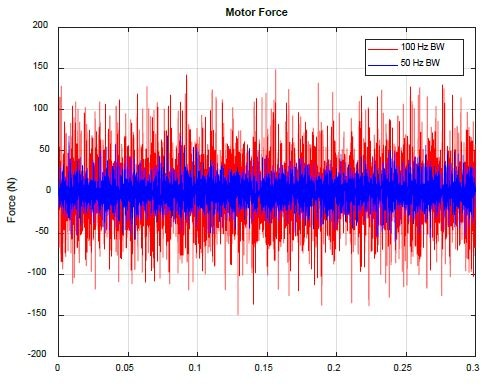Figure 5. Motor force for 50 Hz vs. 100 Hz bandwidth

Figure 6 summarizes the example given above. The net result is that higher bandwidth systems can track higher frequency disturbances. Also, higher frequency disturbances typically mean higher accelerations and hence, higher required motor forces.

As power dissipation goes with the square of motor force, any rise in bandwidth frequency significantly influences motor dissipated power and motor sizing.

The final plot in Figure 6 is the resulting acceleration for three cases of servo bandwidths. The red is for the 50 Hz case, green for the 75 Hz case, and yellow for the 100 Hz case. As can be seen in the plots shown in Figure 6, the RMS of acceleration, and resulting force, is the area under the acceleration spectrum plot, and the smaller the bandwidth, the smaller the RMS acceleration.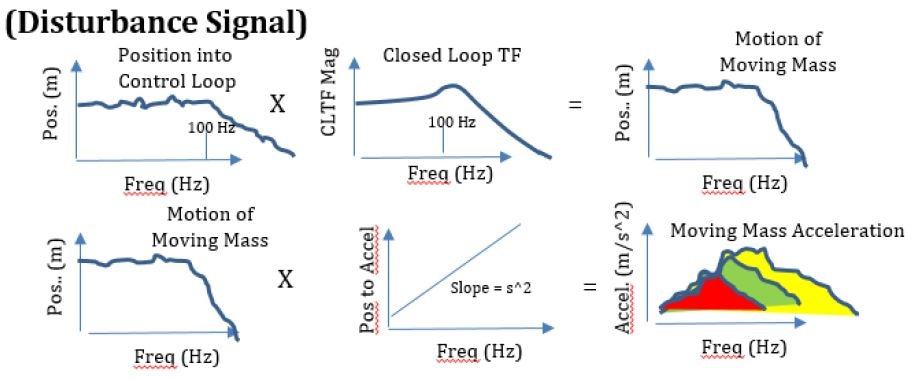Figure 6. Pictorial of analysis

## Case Study Summaries

Two very common, yet different, cases that affect motor sizing are discussed in this article. Case 1 describes when the control system has to move a load from position A to position B and repeat, and Case 2 shows where the control system has to track (or reject) a disturbance signal. In both the cases, the ultimate driving factor is the power dissipated in the motor.

In Case 1, the dissipated power is predominantly a function of the duty cycle of motion, the moving mass (or inertia), and the acceleration achieved during the move. Also, it is independent of servo loop bandwidth. The “backbone” of the motion trajectory in Case 1 is the same independent of servo bandwidth (within the amount of servo tracking error).

However, in Case 2, the servo bandwidth significantly influences the dissipated power. Higher bandwidth servos ask the controller to track higher frequencies, meaning higher accelerations and higher motor forces. In this example, a 2x increase in servo bandwidth produced 6x more dissipated power in the motor.

In Case 1 and Case 2, servo bandwidth is ultimately influenced by servo stability, which means gain margin and phase margin must always be met. In certain cases like Case 2, the servo bandwidth may not be limited by stability, but by allowable dissipated power.

In both cases use the dissipated power to size the motor. The load mass (or inertia), its acceleration, and its duty cycle all collectively have an impact on motor power dissipation.

## Conclusion

The allowable power dissipation in the motor is the driving factor for motor sizing. When selecting a motor, it is necessary to consider how the motor will be used and carry out the appropriate analysis to arrive at the predicted motor power dissipation.

The two cases described in this article require two different types of analysis. In Case 1, the focus area of the analysis is the motion trajectory, whereas in Case 2, the focus area is the servo bandwidth. In both cases, motor power dissipation is driven by the acceleration of the load and its mass.This information has been sourced, reviewed and adapted from materials provided by Celera Motion.

## Citations

• APA

Celera Motion. (2020, October 23). Motion Control – How Are the Motor Size, Power Dissipation and Servo Bandwidth Related?. AZoSensors. Retrieved on November 24, 2020 from https://www.azosensors.com/article.aspx?ArticleID=732.

• MLA

Celera Motion. "Motion Control – How Are the Motor Size, Power Dissipation and Servo Bandwidth Related?". AZoSensors. 24 November 2020. <https://www.azosensors.com/article.aspx?ArticleID=732>.

• Chicago

Celera Motion. "Motion Control – How Are the Motor Size, Power Dissipation and Servo Bandwidth Related?". AZoSensors. https://www.azosensors.com/article.aspx?ArticleID=732. (accessed November 24, 2020).

• Harvard

Celera Motion. 2020. Motion Control – How Are the Motor Size, Power Dissipation and Servo Bandwidth Related?. AZoSensors, viewed 24 November 2020, https://www.azosensors.com/article.aspx?ArticleID=732.